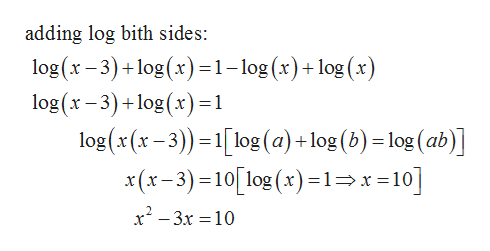# Please solve the following equation and express the solution in exact form:log(x-3) = 1-logxThank you!

Question
10 views

Please solve the following equation and express the solution in exact form:

log(x-3) = 1-logx

Thank you!

check_circle

Step 1

According to the given question it is required to solve the given equation:

Step 2

Now solve the e...help_outlineImage Transcriptioncloseadding log bith sides: log (x-3)log (x)=1-log (x)+log (x log(x-3)+log (x)1 log(x(x-3)-1log (a) +log (b) = log (ab)] x(x-3)-10[ log (x)=1> x -10] x23x 10 fullscreen

### Want to see the full answer?

See Solution

#### Want to see this answer and more?

Solutions are written by subject experts who are available 24/7. Questions are typically answered within 1 hour.*

See Solution
*Response times may vary by subject and question.
Tagged in

### Algebra